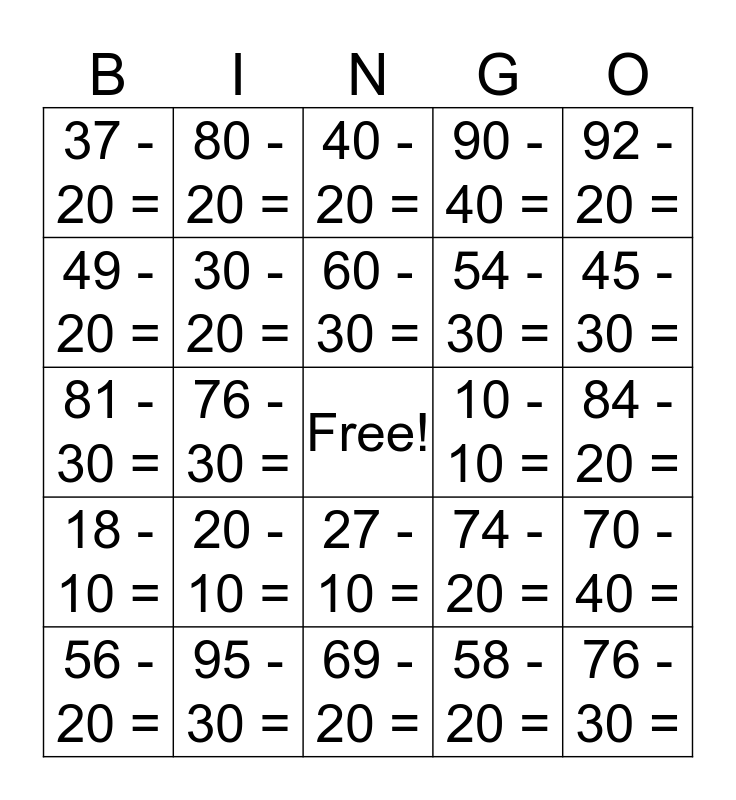# Subtracting Multiples of 10This bingo card has a free space and 29 words: 56 - 20 =, 48 - 10 =, 76 - 30 =, 62 - 10 =, 37 - 20 =, 40 - 20 =, 30 - 20 =, 20 - 10 =, 10 - 10 =, 95 - 30 =, 84 - 20 =, 76 - 30 =, 69 - 20 =, 58 - 20 =, 45 - 30 =, 39 - 10 =, 27 - 10 =, 18 - 10 =, 92 - 20 =, 81 - 30 =, 74 - 20 =, 65 - 30 =, 54 - 30 =, 49 - 20 =, 90 - 40 =, 80 - 20 =, 70 - 40 =, 60 - 30 = and 50 - 20 =.

⚠ This card has duplicate items: 76 - 30 = (2)

## Play Online